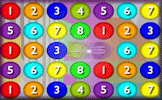# Iteration

## Find approximate solutions to equations numerically using iteration.

##### FibonacciLevel 1Level 2Level 3Level 4Exam-StyleDescriptionHelpMore Algebra

This is level 1: generating sequences using next term rule. You can earn a trophy if you get at least 5 questions correct.

 1. A sequence begins with the number 11 and then each of the following terms is 7 more than the previous term.This can be written as $$x_n = x_{n-1} + 7$$ where $$x_1 = 11$$.What is the second term of this sequence?2. Find the third term of the sequence given by $$x_n = x_{n-1} + 5$$The first term of the sequence $$x_1 = 13$$.3. Find the fourth term of the sequence given by $$x_n = x_{n-1} - 6$$The first term of the sequence $$x_1 = 15$$.4. Find the fifth term of the sequence given by $$x_n = 2x_{n-1} + 1$$The first term of the sequence $$x_1 = 2$$.5. Find the fifth term of the sequence given by $$x_n = 2x_{n-1}^2 + 2$$The third term of the sequence $$x_3 = 3$$.6. Find the first term of the sequence given by $$x_n = 5x_{n-1} - 6$$The third term of the sequence $$x_3 = 439$$.Check

This is Iteration level 1. You can also try:
Level 2 Level 3 Level 4

## Instructions

Try your best to answer the questions above. Type your answers into the boxes provided leaving no spaces. As you work through the exercise regularly click the "check" button. If you have any wrong answers, do your best to do corrections but if there is anything you don't understand, please ask your teacher for help.

When you have got all of the questions correct you may want to print out this page and paste it into your exercise book. If you keep your work in an ePortfolio you could take a screen shot of your answers and paste that into your Maths file.

## Transum.org

This web site contains over a thousand free mathematical activities for teachers and pupils. Click here to go to the main page which links to all of the resources available.## More Activities:

Mathematicians are not the people who find Maths easy; they are the people who enjoy how mystifying, puzzling and hard it is. Are you a mathematician?

Comment recorded on the 12 July 'Starter of the Day' page by Miss J Key, Farlingaye High School, Suffolk:

"Thanks very much for this one. We developed it into a whole lesson and I borrowed some hats from the drama department to add to the fun!"

Comment recorded on the 1 February 'Starter of the Day' page by M Chant, Chase Lane School Harwich:

"My year five children look forward to their daily challenge and enjoy the problems as much as I do. A great resource - thanks a million."

#### Number Crunch SagaA lively numeracy game requiring you to align three numbers to create the given target sum or product. There are five levels to this online game and a virtual Transum Trophy available for each level.

There are answers to this exercise but they are available in this space to teachers, tutors and parents who have logged in to their Transum subscription on this computer.

A Transum subscription unlocks the answers to the online exercises, quizzes and puzzles. It also provides the teacher with access to quality external links on each of the Transum Topic pages and the facility to add to the collection themselves.

Subscribers can manage class lists, lesson plans and assessment data in the Class Admin application and have access to reports of the Transum Trophies earned by class members.

Subscribe

## Go Maths

Learning and understanding Mathematics, at every level, requires learner engagement. Mathematics is not a spectator sport. Sometimes traditional teaching fails to actively involve students. One way to address the problem is through the use of interactive activities and this web site provides many of those. The Go Maths page is an alphabetical list of free activities designed for students in Secondary/High school.

## Maths Map

Are you looking for something specific? An exercise to supplement the topic you are studying at school at the moment perhaps. Navigate using our Maths Map to find exercises, puzzles and Maths lesson starters grouped by topic.

## Teachers

If you found this activity useful don't forget to record it in your scheme of work or learning management system. The short URL, ready to be copied and pasted, is as follows:

Do you have any comments? It is always useful to receive feedback and helps make this free resource even more useful for those learning Mathematics anywhere in the world. Click here to enter your comments.For All:

## Description of Levels

CloseFibonacci Probably the first iterative example learners will encounter is the Finonacci sequence. A simple rule exists for finding the next term of the sequence from the previous two.

Level 1 - Generating sequences using next term rule.

Level 2 - Rearranging equations.

Level 3 - Using flowcharts to define iterations.

Level 4 - Solving equations to one decimal place.

Exam Style questions are in the style of GCSE or IB/A-level exam paper questions and worked solutions are available for Transum subscribers.

Answers to this exercise are available lower down this page when you are logged in to your Transum account. If you don’t yet have a Transum subscription one can be very quickly set up if you are a teacher, tutor or parent.

## Example

Don't wait until you have finished the exercise before you click on the 'Check' button. Click it often as you work through the questions to see if you are answering them correctly.

For an animated demonstration of the calculator button pressing order for iteration see Calculator Workout skill 16.

Answers to this exercise are available lower down this page when you are logged in to your Transum account. If you don’t yet have a Transum subscription one can be very quickly set up if you are a teacher, tutor or parent.

Close